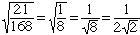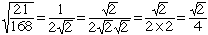grade 11 student can you show me how to do this square root of 21/168 Hi, First you should simplify the fraction 21/168 to get 21/168 = 1/8. 8 is 2x2x2 = 4x2 so the square root of 8 is 2 times the square root of 2. HenceSome teachers don't like to see a root in the denominator of a fraction. If this is true for your teacher then multiply the numerator and denominator by the square root of 2. This givesCheers, Penny Go to Math Central